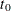### Outlier Detection

In time series analysis it is often useful to detect changes over time in the characteristics of the response series. In the UCM procedure you can search for two types of changes, additive outliers (AO) and level shifts (LS). An additive outlier is an unusual value in the series, the cause of which might be a data recording error or a temporary shock to the series generation process. A level shift represents a permanent shift, either up or down, in the level of the series. You can control different aspects of the outlier search, such as the significance level of the reported outliers, by choosing different options in the OUTLIER statement. The search for AOs is done by default, whereas the CHECKBREAK option in the LEVEL statement must be used to turn on the search for LSs.

The outlier detection process implemented in the UCM procedure is based on de Jong and Penzer (1998). In this approach the fitted model is taken to be the null model, and the series values and level shifts that are not adequately accounted for by the null model are flagged as outliers. The unusualness of a response series value at a particular time point, with respect to the fitted model, can be judged by estimating its value based on the rest of the data (that is, the series obtained by deleting the series value at) and comparing the estimated value to the observed value. If the difference between the estimated and observed values is statistically significant, then such value can be regarded as an AO. Note that this difference between the estimated and observed values is also the regression coefficient of a dummy regressor that takes the value 1.0 atand is 0.0 elsewhere, assuming such a regressor is added to the null model. In this way the series value atis regarded as AO if the regression coefficient of this dummy regressor is significant. Similarly, you can say that a level shift has occurred at a time pointif the regression coefficient of a regressor, which is 0.0 beforeand 1.0 atand thereafter, is statistically significant. De Jong and Penzer (1998) provide an efficient way to compute such AO and LS regression coefficients and their standard errors at all time points in the series. The outlier summary table, which is produced by default, simply lists the most statistically significant candidates among these.